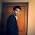# How to deal insignificant levels of a categorical variable

This tutorial describes how to interpret or treat insignificant levels of a independent categorical variable in a regression (linear or logistic) model. It is one of the most frequently asked question in predictive modeling.

Case Study
Suppose you are building a linear (or logistic) regression model. In your independent variables list, you have a categorical variable with 4 categories (or levels). You created 3 dummy variables (k-1 categories) and set one of the category as a reference category. Then you run stepwise / backward/ forward regression technique and you found only one of the category coming out statistically significant based on p-value and the remaining 3 categories are insignificant. The question arises - should we remove or keep these 3 categories having insignificant difference? should we include the whole categorical variable or not?

Solution
In short, the answer is we can ONLY choose whether we should use this independent categorical variable as a whole or not. In other words, we should only see whether the categorical variable as a whole is significant or not. We cannot include some categories of a variable and exclude some categories having insignificant difference.

Why we cannot choose categories of a variable

Suppose you have a nominal categorical variable having 4 categories (or levels). You would create 3 dummy variables (k-1 = 4-1 dummy variables) and set one category as a reference level. Suppose one of them is insignificant.  Then if you exclude that dummy variable, it would change the reference level as you are indirectly combining that insignificant level with the original reference level. It would have a new reference level and interpretation would change. Moreover, excluding the level may make the others insignificant.

How it works

Suppose you have 2 continuous independent variables - GRE (Graduate Record Exam scores), GPA (grade point average) and 1 categorical independent variable- RANK (prestige of the undergraduate institution and levels ranging from 1 through 4. Institutions with a rank of 1 have the highest prestige, while those with a rank of 4 have the lowest ). Dependent variable - ADMIT (admission into graduate school)

First Step,  3 dummy variables are entered into the model as set as (K-1) dummy variables where K=4 is the number of categories in the variable 'rank'.

Run Model
# Read and prepare data
dt\$rank <- as.factor(mydata\$rank)

# First Model (Including 'rank')
logit <- glm(admit ~ gre + gpa + rank, data = dt, family = "binomial")

#Summary of first model
summary(logit)

Interpretation
The category (or level) 1 of 'rank' variable has been set reference category and coefficient of rank2 means the difference between the coefficient of rank1 and the rank2. The p-value tells us whether the difference between the coefficient of the rank1 and the rank2 differs from zero. In this case, it is statistically significant from 0 as p-value is less than 0.05. The same interpretation holds for other 2 categories - rank3 and rank4.

Strategy : Build 2 Models (With and Without the categorical variable)

We can make decision about inclusion of the variable by building 2 models -with or without the variable and then check a likelihood ratio test.
# Second Model (Excluding 'rank')
logit2 <- glm(admit ~ gre + gpa, data = dt, family = "binomial")
#Summary of second model
summary(logit2)

Likelihood Ratio Test

It is performed by estimating two models and comparing the fit of one model to the fit of the other. Removing predictor variables from a model will almost always make the model fit less well but it is necessary to test whether the observed difference in model fit is statistically significant. It tests whether this difference is statistically significant.
#Likelihood Ratio Test
anova(logit, logit2, test="LRT")Likelihood Ratio Test Results
Since p-value is less than 0.05, it means the difference is significant and the variable 'rank' should be included in the model.

We can further calculate AUC (Area under curve) of both the models.
#Prediction - First Model
pred = predict(logit,dt, type = "response")
#Prediction - Second Model
pred2 = predict(logit2, dt, type = "response")
#Storing Model Performance Scores
library(ROCR)
# Calculating Area under Curve - First Model
perf <- performance(prediction(pred ,dt\$admit),"auc")
perf
# Calculating Area under Curve - Second Model
perf2 <- performance(prediction(pred2 ,dt\$admit),"auc")
perf2
The AUC score of the first model (including rank) is 0.6928 and the AUC of the other model is 0.6354. It shows first model fits the model well.

How about combining categories?

There is no clear-cut answer. Sometimes, it makes sense to combine categories and use it in the model. It all depends on the nature and physical meaning of the variable. Sometimes, it does not make sense to combine the categories.Related PostsDeepanshu founded ListenData with a simple objective - Make analytics easy to understand and follow. He has over 8 years of experience in data science. During his tenure, he has worked with global clients in various domains like Banking, Insurance, Telecom and Human Resource.

4 Responses to "How to deal insignificant levels of a categorical variable"
1.Maybe the WoE transformation on this variable will avoid such situation?

1.WOE transformation does not exist for linear regression. It's not always a solution when there is a categorical variable.

2.Hi,
I have 136 predictor variables, among which 130 variable has only one level and 6 variables has 2 levels. Can I do step wise regression with this kind of data? If not, can you explain me why it is not possible to do?
Thank you

3.Can WOE transformation of a continuous variable be used in logistic regression instead of creating dummy variables?

Next → ← Prev
Love this Post? Spread the Word!
Share